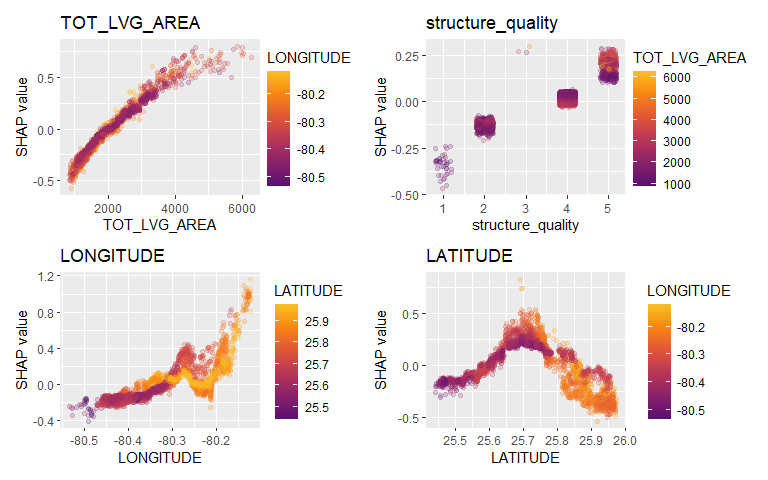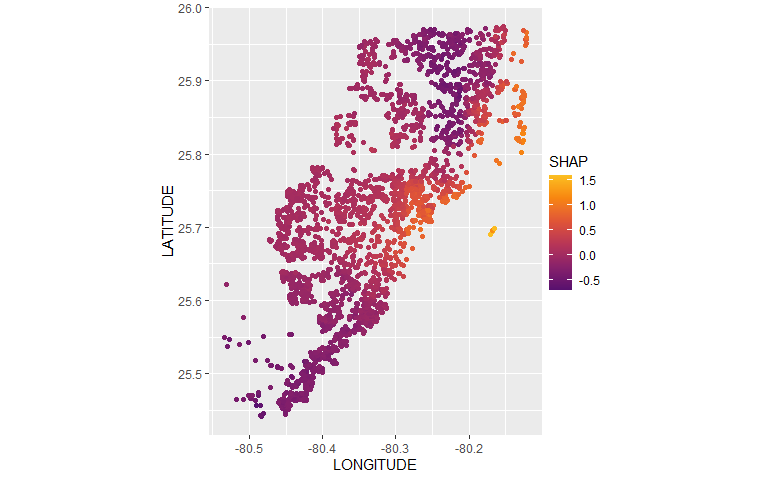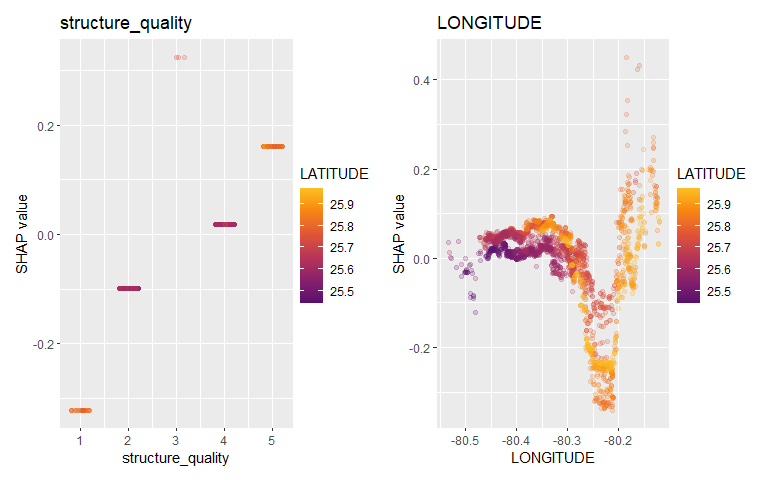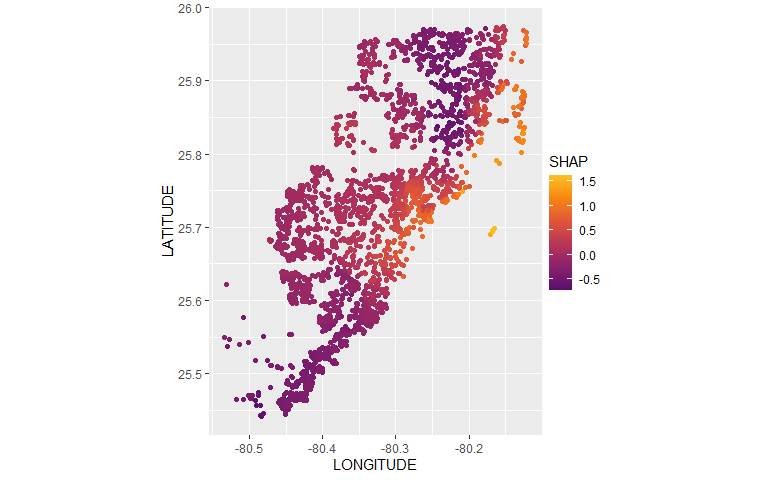# Geographic Components

## Setting

In a model with geographic components, we want to express a functional $$T$$ (usually the expectation or a quantile) of a response $$Y$$ as a function $$f$$ of a set of geographic features (latitude/longitude and/or postal code and/or other features varying with location), and other features:

$T(Y \mid X^\textrm{geo}, X^\textrm{other}) \approx f(X^\textrm{geo}, X^\textrm{other})$ Like any feature, the effect of a single geographic feature $$X^{\textrm{geo}, j}$$ can be described using SHAP dependence plots. However, studying the effect of latitude (or any other location dependent feature) alone is often not very illuminating - simply due to strong interaction effects and correlations with other geographic features.

That’s where the additivity of SHAP values comes into play: The sum of SHAP values of all geographic components represent the total effect of $$X^\textrm{geo}$$, and this sum can be visualized as a heatmap or 3D scatterplot against latitude/longitude (or any other geographic representation)

## A first example

For illustration, we will use a beautiful house price dataset containing information on about 14’000 houses sold in 2016 in Miami-Dade County. Some of the columns are as follows:

• SALE_PRC: Sale price in USD: Its logarithm will be our model response.
• LATITUDE, LONGITUDE: Coordinates
• CNTR_DIST: Distance to central business district
• OCEAN_DIST: Distance (ft) to the ocean
• RAIL_DIST: Distance (ft) to the next railway track
• HWY_DIST: Distance (ft) to next highway
• TOT_LVG_AREA: Living area in square feet
• LND_SQFOOT: Land area in square feet
• structure_quality: Measure of building quality (1: worst to 5: best)
• age: Age of the building in years

(Italic features are geographic components.) For more background on this dataset, see Mayer et al. (2022).

We will fit an XGBoost model to explain log(price) as a function of lat/long, size, and quality/age.

library(xgboost)
library(ggplot2)
library(shapviz)

#>   LATITUDE LONGITUDE     PARCELNO SALE_PRC LND_SQFOOT TOT_LVG_AREA
#> 0 25.89103 -80.16056 622280070620   440000       9375         1753
#> 1 25.89132 -80.15397 622280100460   349000       9375         1715
#> 2 25.89133 -80.15374 622280100470   800000       9375         2276
#> 3 25.89176 -80.15266 622280100530   988000      12450         2058
#> 4 25.89182 -80.15464 622280100200   755000      12800         1684
#> 5 25.89206 -80.16135 622280070180   630000       9900         1531
#>   SPEC_FEAT_VAL RAIL_DIST OCEAN_DIST WATER_DIST CNTR_DIST SUBCNTR_DI HWY_DIST
#> 0             0    2815.9    12811.4      347.6   42815.3    37742.2  15954.9
#> 1             0    4359.1    10648.4      337.8   43504.9    37340.5  18125.0
#> 2         49206    4412.9    10574.1      297.1   43530.4    37328.7  18200.5
#> 3         10033    4585.0    10156.5        0.0   43797.5    37423.2  18514.4
#> 4         16681    4063.4    10836.8      326.6   43599.7    37550.8  17903.4
#> 5          2978    2391.4    13017.0      188.9   43135.1    38176.2  15687.2
#>   age avno60plus month_sold structure_quality
#> 0  67          0          8                 4
#> 1  63          0          9                 4
#> 2  61          0          2                 4
#> 3  63          0          9                 4
#> 4  42          0          7                 4
#> 5  41          0          2                 4

x_coord <- c("LATITUDE", "LONGITUDE")
x_nongeo <- c("TOT_LVG_AREA", "LND_SQFOOT", "structure_quality", "age")
x <- c(x_coord, x_nongeo)

# Train/valid split
set.seed(1)
ix <- sample(nrow(miami), 0.8 * nrow(miami))
X_train <- data.matrix(miami[ix, x])
X_valid <- data.matrix(miami[-ix, x])
y_train <- log(miami$SALE_PRC[ix]) y_valid <- log(miami$SALE_PRC[-ix])

# Fit XGBoost model with early stopping
dtrain <- xgb.DMatrix(X_train, label = y_train)
dvalid <- xgb.DMatrix(X_valid, label = y_valid)

params <- list(learning_rate = 0.2, objective = "reg:squarederror", max_depth = 5)

fit <- xgb.train(
params = params,
data = dtrain,
watchlist = list(valid = dvalid),
early_stopping_rounds = 20,
nrounds = 1000,
callbacks = list(cb.print.evaluation(period = 100))
)
#>   valid-rmse:9.784245
#> Will train until valid_rmse hasn't improved in 20 rounds.
#>
#>     valid-rmse:0.152615
#>     valid-rmse:0.149175
#> Stopping. Best iteration:
#>     valid-rmse:0.148793

Let’s first study selected SHAP dependence plots, evaluated on the validation dataset with around 2800 observations. Note that we could as well use the training data for this purpose, but it is a bit too large.

sv <- shapviz(fit, X_pred = X_valid)
sv_dependence(
sv,
v = c("TOT_LVG_AREA", "structure_quality", "LONGITUDE", "LATITUDE"),
alpha = 0.2
)# And now the two-dimensional plot of the sum of SHAP values
sv_dependence2D(sv, x = "LONGITUDE", y = "LATITUDE") +
coord_equal()The last plot gives a good impression on price levels.

Notes:

1. Since we have modeled logarithmic prices, the effects are on relative scale (0.1 means about 10% above average).
2. Due to interaction effects with non-geographic components, the location effects might depend on features like living area. This is not visible in above plot. We will modify the model now in this respect.

## Two modifications

We will now change above model in two ways, not unlike the model in Mayer et al. (2022):

1. We will use additional geographic features like distance to railway track or to the ocean.
2. We will use interaction constraints to allow only interactions between geographic features.

The second step leads to a model that is additive in each non-geographic component and also additive in the combined location effect. According to the technical report Mayer (2022), SHAP dependence plots of additive components in a boosted trees model are shifted versions of corresponding partial dependence plots (evaluated at observed values). This allows a “Ceteris Paribus” interpretation of SHAP dependence plots of corresponding components.

# Extend the feature set
more_geo <- c("CNTR_DIST", "OCEAN_DIST", "RAIL_DIST", "HWY_DIST")
x2 <- c(x, more_geo)

X_train2 <- data.matrix(miami[ix, x2])
X_valid2 <- data.matrix(miami[-ix, x2])

dtrain2 <- xgb.DMatrix(X_train2, label = y_train)
dvalid2 <- xgb.DMatrix(X_valid2, label = y_valid)

# Build interaction constraint vector
ic <- c(
list(which(x2 %in% c(x_coord, more_geo)) - 1),
as.list(which(x2 %in% x_nongeo) - 1)
)

# Modify parameters
params\$interaction_constraints <- ic

fit2 <- xgb.train(
params = params,
data = dtrain2,
watchlist = list(valid = dvalid2),
early_stopping_rounds = 20,
nrounds = 1000,
callbacks = list(cb.print.evaluation(period = 100))
)
#>   valid-rmse:9.784974
#> Will train until valid_rmse hasn't improved in 20 rounds.
#>
#>     valid-rmse:0.161874
#>     valid-rmse:0.156760
#> Stopping. Best iteration:
#>     valid-rmse:0.155253

# SHAP analysis
sv2 <- shapviz(fit2, X_pred = X_valid2)

# Two selected features: Thanks to additivity, structure_quality can be read as
# Ceteris Paribus
sv_dependence(sv2, v = c("structure_quality", "LONGITUDE"), alpha = 0.2)# Total geographic effect (Ceteris Paribus thanks to additivity)
sv_dependence2D(sv2, x = "LONGITUDE", y = "LATITUDE", add_vars = more_geo) +
coord_equal()Again, the resulting total geographic effect looks reasonable. Note that, unlike in the first example, there are no interactions to non-geographic components, leading to a Ceteris Paribus interpretation.